Updating search results...

# 7 Results

View
Selected filters:
• UT.MATH.I.G.CO.5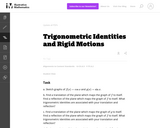Unrestricted Use
CC BY
Rating
0.0 stars

This is a task from the Illustrative Mathematics website that is one part of a complete illustration of the standard to which it is aligned. Each task has at least one solution and some commentary that addresses important asects of the task and its potential use. Here are the first few lines of the commentary for this task: Sketch graphs of $f(x) = \cos{x}$ and $g(x) = \sin{x}$. Find a translation of the plane which maps the graph of $f(x)$ to itself. Find a reflection of ...

Subject:
Mathematics
Secondary Mathematics
Material Type:
Activity/Lab
Provider:
Illustrative Mathematics
Provider Set:
Illustrative Mathematics
Author:
Illustrative Mathematics
01/06/2014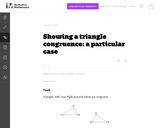Unrestricted Use
CC BY
Rating
0.0 stars

This is a task from the Illustrative Mathematics website that is one part of a complete illustration of the standard to which it is aligned. Each task has at least one solution and some commentary that addresses important asects of the task and its potential use. Here are the first few lines of the commentary for this task: Triangles $ABC$ and $PQR$ pictured below are congruent: Show the congruence using rigid motions of the plane. Can the congruence be shown with a single...

Subject:
Mathematics
Secondary Mathematics
Material Type:
Activity/Lab
Provider:
Illustrative Mathematics
Provider Set:
Illustrative Mathematics
Author:
Illustrative Mathematics
10/30/2013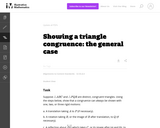Unrestricted Use
CC BY
Rating
0.0 stars

This is a task from the Illustrative Mathematics website that is one part of a complete illustration of the standard to which it is aligned. Each task has at least one solution and some commentary that addresses important asects of the task and its potential use. Here are the first few lines of the commentary for this task: Suppose $\triangle ABC$ and $\triangle PQR$ are distinct, congruent triangles. Using the steps below, show that a congruence can always be shown with o...

Subject:
Mathematics
Secondary Mathematics
Material Type:
Activity/Lab
Provider:
Illustrative Mathematics
Provider Set:
Illustrative Mathematics
Author:
Illustrative Mathematics
10/29/2013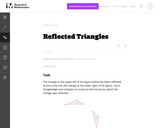Unrestricted Use
CC BY
Rating
0.0 stars

This task is a reasonably straight-forward application of rigid motion geometry, with emphasis on ruler and straightedge constructions, and would be suitable for assessment purposes.

Subject:
Mathematics
Secondary Mathematics
Material Type:
Activity/Lab
Provider:
Illustrative Mathematics
Provider Set:
Illustrative Mathematics
Author:
Illustrative Mathematics
05/01/2012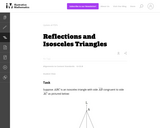Unrestricted Use
CC BY
Rating
0.0 stars

This activity is one in a series of tasks using rigid transformations of the plane to explore symmetries of classes of triangles, with this task in particular focussing on the class of isosceles triangles.

Subject:
Mathematics
Secondary Mathematics
Material Type:
Activity/Lab
Provider:
Illustrative Mathematics
Provider Set:
Illustrative Mathematics
Author:
Illustrative Mathematics
01/05/2013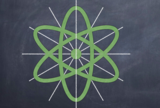Conditional Remix & Share Permitted
CC BY-NC
Rating
0.0 stars

This is a lesson plan that introduces rotational and reflectional symmetry with geometric shapes, focusing on regular polygons. Notes (two copies: a student version and also an answer key, both as a Word doc and a PDF), assignment, and cut-out shapes are provided. There is a final project, with both instructions and an example included. Cover image: Christensen, K., 2022. Reflectional Symmetry of an Atom [screenshot] (Keynote presentation, Rotational and Reflectional Symmetry Assignment).

Subject:
Secondary Mathematics
Material Type:
Activity/Lab
Assessment
Diagram/Illustration
Homework/Assignment
Lesson Plan
Author:
Kimberly
03/09/2022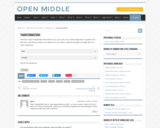Restricted Use